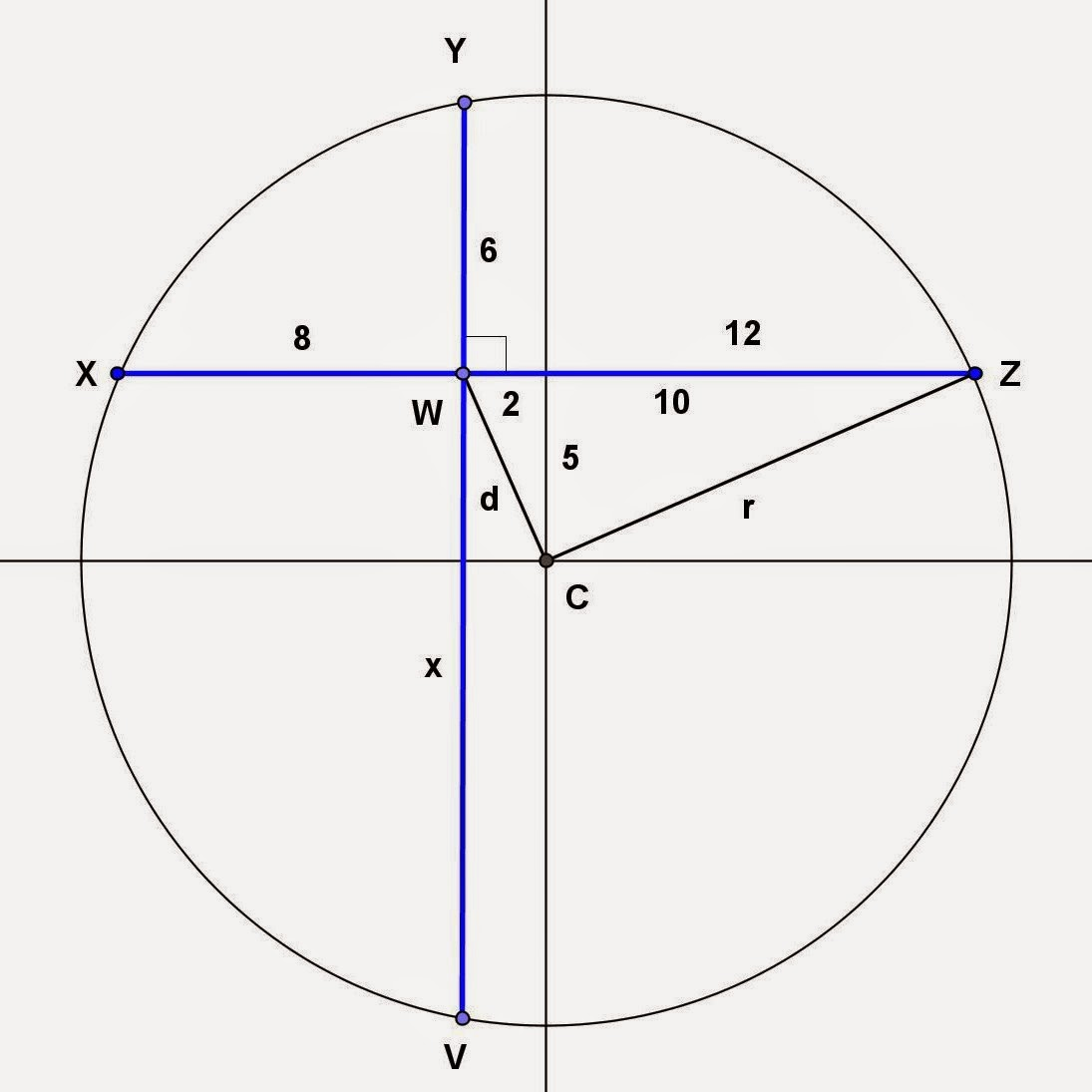## Thursday, December 18, 2014

### Circle and Secant Segment Problems, 4

Category: Plane Geometry

"Published in Newark, California, USA"

A circle can be drawn through points X, Y, and Z.
a. What is the radius of the circle?
b. How far is the center of the circle from point W?Photo by Math Principles in Everyday Life

Solution:

Consider the given figure abovePhoto by Math Principles in Everyday Life

Expand the line segment YW from point W and label the other point as point V.Photo by Math Principles in Everyday Life

If a theorem says "When two chords intersect inside a circle, the product of the segments of one chord equals the product of the segments of the other chord.", then the working equation is

Hence, the length of XZ is 20 and YV is 22. The midpoint of XZ is 10 units from X and the midpoint of YV is 11 units from Y. From their midpoints, draw a vertical and a horizontal line to locate their intersection which is point C as the center of a circle as followsPhoto by Math Principles in Everyday Life

From the figure, by using Pythagorean Theorem, the distance of C from W is

and the radius of a circle which is the distance of C from Z is

If you will get the distance of C from X, Y, or V, the value of length must be the same otherwise point C is not the center of a circle.Photo by Math Principles in Everyday Life